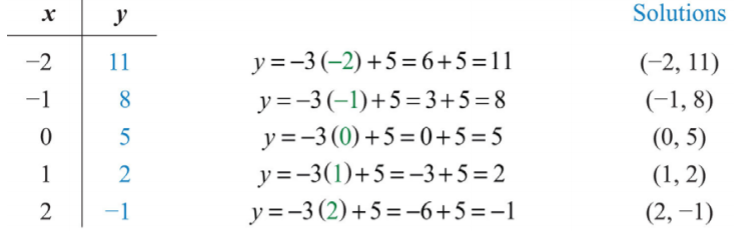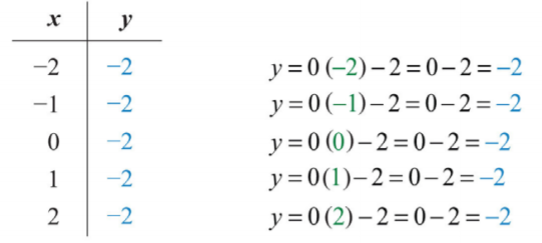# 3.2: Graph by Plotting Points

•• Anonymous
• LibreTexts
$$\newcommand{\vecs}{\overset { \rightharpoonup} {\mathbf{#1}} }$$ $$\newcommand{\vecd}{\overset{-\!-\!\rightharpoonup}{\vphantom{a}\smash {#1}}}$$$$\newcommand{\id}{\mathrm{id}}$$ $$\newcommand{\Span}{\mathrm{span}}$$ $$\newcommand{\kernel}{\mathrm{null}\,}$$ $$\newcommand{\range}{\mathrm{range}\,}$$ $$\newcommand{\RealPart}{\mathrm{Re}}$$ $$\newcommand{\ImaginaryPart}{\mathrm{Im}}$$ $$\newcommand{\Argument}{\mathrm{Arg}}$$ $$\newcommand{\norm}{\| #1 \|}$$ $$\newcommand{\inner}{\langle #1, #2 \rangle}$$ $$\newcommand{\Span}{\mathrm{span}}$$ $$\newcommand{\id}{\mathrm{id}}$$ $$\newcommand{\Span}{\mathrm{span}}$$ $$\newcommand{\kernel}{\mathrm{null}\,}$$ $$\newcommand{\range}{\mathrm{range}\,}$$ $$\newcommand{\RealPart}{\mathrm{Re}}$$ $$\newcommand{\ImaginaryPart}{\mathrm{Im}}$$ $$\newcommand{\Argument}{\mathrm{Arg}}$$ $$\newcommand{\norm}{\| #1 \|}$$ $$\newcommand{\inner}{\langle #1, #2 \rangle}$$ $$\newcommand{\Span}{\mathrm{span}}$$$$\newcommand{\AA}{\unicode[.8,0]{x212B}}$$

Learning Objectives

• Verify solutions to linear equations with two variables.
• Graph lines by plotting points.
• Identify and graph horizontal and vertical lines.

## Solutions to Equations with Two Variables

A linear equation with two variables has standard form $$ax+by=c$$, where $$a,b$$, and $$c$$ are real numbers and $$a$$ and $$b$$ are not both $$0$$. Solutions to equations of this form are ordered pairs $$(x, y)$$, where the coordinates, when substituted into the equation, produce a true statement.

Example $$\PageIndex{1}$$

Determine whether $$(1, −2)$$ and $$(−4, 1)$$ are solutions to $$6x−3y=12$$.

Solution:

Substitute the $$x$$- and $$y$$-values into the equation to determine whether the ordered pair produces a true statement.

$$\begin{array}{c|c}{\underline{Check\:\:(1,-2)}}&{\underline{Check\:\:(-4,1)}}\\{6x-3y=12}&{6x-3y=12} \\{6(\color{OliveGreen}{1}\color{black}{)-3(}\color{OliveGreen}{-2}\color{black}{)=12}}&{6(\color{OliveGreen}{-4}\color{black}{)-3(}\color{OliveGreen}{1}\color{black}{)=12}}\\{6+6=12}&{-24-3=12}\\{12=12\quad\color{Cerulean}{\checkmark}}&{-27=12\quad\color{red}{x}}\end{array}$$

$$(1,-2)$$ is a solution, and $$(-4,1)$$ is not.

It is often the case that a linear equation is given in a form where one of the variables, usually $$y$$, is isolated. If this is the case, then we can check that an ordered pair is a solution by substituting in a value for one of the coordinates and simplifying to see if we obtain the other.

Example $$\PageIndex{2}$$

Are $$(\frac{1}{2}, −3)$$ and $$(−5, 14)$$ solutions to $$y=2x−4$$?

Solution:

Substitute the $$x$$-values and simplify to see if the corresponding $$y$$-values are obtained.

$$\begin{array}{c|c} {\underline{x=\frac{1}{2}}}&{\underline{x=-5}}\\{y=2x-4}&{y=2x-4}\\{=2\color{black}{\left(\color{OliveGreen}{\frac{1}{2}} \right)-4}}&{=2(\color{OliveGreen}{-5}\color{black}{)-4}}\\{=1-4}&{=-10-4}\\{=-3\quad\color{Cerulean}{\checkmark}}&{=-14\quad\color{red}{x}} \end{array}$$

$$(\frac{1}{2},-3)$$ is a solution, and $$(-5,14)$$ is not.

Exercise $$\PageIndex{1}$$

Is $$(6, −1)$$ a solution to $$y=−\frac{2}{3}x+3$$?

Yes

When given linear equations with two variables, we can solve for one of the variables, usually $$y$$, and obtain an equivalent equation as follows:

Written in this form, we can see that $$y$$ depends on $$x$$. Here $$x$$ is the independent variable and $$y$$ is the dependent variable.

\left. \begin{aligned} 6x-3y&=12 \\ y&=2x-4\end{aligned}\right\} \color{Cerulean}{Equivalent\:equations}

The linear equation $$y=2x−4$$ can be used to find ordered pair solutions. If we substitute any real number for $$x$$, then we can simplify to find the corresponding y-value. For example, if $$x=3$$, then $$y=2(3)−4=6−4=2$$, and we can form an ordered pair solution, $$(3, 2)$$. Since there are infinitely many real numbers to choose for $$x$$, the linear equation has infinitely many ordered pair solutions $$(x, y)$$.

Example $$\PageIndex{3}$$

Find ordered pair solutions to the equation $$5x−y=14$$ with the given $$x$$-values $$\{−2, −1, 0, 4, 6\}$$.

Solution:

First, solve for $$y$$.

Next, substitute the $$x$$-values in the equation $$y=5x−14$$ to find the corresponding $$y$$-values.

$$x-value$$ $$y-value$$ $$Solution$$ $$x=-2$$ $$(-2,-24)$$ $$x=-1$$ $$(-1,-19)$$ $$x=0$$ $$(0,-14)$$ $$x=4$$ $$(4,6)$$ $$x=6$$ $$(6,16)$$ Table $$\PageIndex{1}$$

$$\{(−2, −24), (−1, −19), (0, −14), (4, 6), (6, 16)\}$$

In the previous example, certain $$x$$-values are given, but that is not always going to be the case. When treating $$x$$ as the independent variable, we can choose any values for $$x$$ and then substitute them into the equation to find the corresponding $$y$$-values. This method produces as many ordered pair solutions as we wish.

Example $$\PageIndex{4}$$

Find five ordered pair solutions to $$6x+2y=10$$.

Solution:

First, solve for $$y$$.

\begin{aligned} 6x+2y&=10 \\ 6x+2y\color{Cerulean}{-6x}&=10\color{Cerulean}{-6x} \\ 2y&=-6x+10 \\ \frac{2y}{\color{Cerulean}{2}}&=\frac{-6x+10}{\color{Cerulean}{2}} \\ y&=\frac{-6x}{2}+\frac{10}{2} \\ y&=-3x+5 \end{aligned}

Next, choose any set of $$x$$-values. Usually we choose some negative values and some positive values. In this case, we will find the corresponding $$y$$-values when $$x$$ is $$\{−2, −1, 0, 1, 2\}$$. Make the substitutions required to fill in the following table (often referred to as a t-chart):Figure $$\PageIndex{1}$$

$$\{(−2, 11), (−1, 8), (0, 5), (1, 2), (2, −1)\}$$. Since there are infinitely many ordered pair solutions, answers may vary depending on the choice of values for the independent variable.

Exercise $$\PageIndex{2}$$

Find five ordered pair solutions to $$10x−2y=2$$.

$$\{(−2, −11), (−1, −6), (0, −1), (1, 4), (2, 9)\}$$ (answers may vary)

## Graph by Plotting Points

Since the solutions to linear equations are ordered pairs, they can be graphed using the rectangular coordinate system. The set of all solutions to a linear equation can be represented on a rectangular coordinate plane using a straight line connecting at least two points; this line is called its graph. To illustrate this, plot five ordered pair solutions, $$\{(−2, 11), (−1, 8), (0, 5), (1, 2), (2, −1)\}$$, to the linear equation $$6x+2y=10$$.Figure $$\PageIndex{2}$$

Notice that the points are collinear; this will be the case for any linear equation. Draw a line through the points with a straightedge, and add arrows on either end to indicate that the graph extends indefinitely.Figure $$\PageIndex{3}$$

The resulting line represents all solutions to $$6x+2y=10$$, of which there are infinitely many. The steps for graphing lines by plotting points are outlined in the following example.

Example $$\PageIndex{5}$$

Find five ordered pair solutions and graph:

$$10x−5y=10$$.

Solution:

Step 1: Solve for $$y$$.

Step 2: Choose at least two $$x$$-values and find the corresponding $$y$$-values. In this section, we will choose five real numbers to use as $$x$$-values. It is a good practice to choose $$0$$ and some negative numbers, as well as some positive numbers.Figure $$\PageIndex{4}$$

It will not always be the case that $$y$$ can be solved in terms of $$x$$ with integer coefficients. In fact, the coefficients often turn out to be fractions.

Example $$\PageIndex{6}$$

Find five ordered pair solutions and graph:

$$−5x+2y=10$$.

Solution:

\begin{aligned} -5x+2y&=10 \\ -5x+2y\color{Cerulean}{+5x}&=10\color{Cerulean}{+5x} \\ 2y&=5x+10 \\ frac{2y}{\color{Cerulean}{2}}&=\frac{5x+10}{\color{Cerulean}{2}} \\ y&=\frac{5x}{2}+\frac{10}{2} \\ y&=\frac{5}{2}x+5 \end{aligned}

Remember that you can choose any real number for the independent variable $$x$$, so choose wisely here. Since the denominator of the coefficient of the variable $$x$$ is $$2$$, you can avoid fractions by choosing multiples of $$2$$ for the $$x$$-values. In this case, choose the set of $$x$$-values $$\{−6, −4, −2, 0, 2\}$$ and find the corresponding $$y$$-values.Figure $$\PageIndex{6}$$

Five solutions are $$\{(−6, −10), (−4, −5), (−2, 0), (0, 5), (2, 10)\}$$. Here we choose to scale the $$x$$-axis with multiples of $$2$$ and the $$y$$-axis with multiples of $$5$$.Figure $$\PageIndex{7}$$

Exercise $$\PageIndex{3}$$

Find five ordered pair solutions and graph: $$x+2y=6$$.

$$\{(−2, 4), (0, 3), (2, 2), (4, 1), (6, 0)\}$$Figure $$\PageIndex{8}$$

## Horizontal and Vertical Lines

We need to recognize by inspection linear equations that represent a vertical or horizontal line.

Example $$\PageIndex{7}$$

Graph by plotting five points: $$y=−2$$.

Solution:

Since the given equation does not have a variable $$x$$, we can rewrite it with a $$0$$ coefficient for $$x$$.

$$y=0x-2$$

Choose any five values for $$x$$ and see that the corresponding $$y$$-value is always $$−2$$.Figure $$\PageIndex{9}$$

We now have five ordered pair solutions to plot $$\{(−2, −2), (−1, −2), (0, −2), (1, −2), (2, −2)\}$$.Figure $$\PageIndex{10}$$

When the coefficient for the variable $$x$$ is $$0$$, the graph is a horizontal line. In general, the equation for a horizontal line can be written in the form $$y=k$$, where $$k$$ represents any real number.

Example $$\PageIndex{8}$$

Graph by plotting five points: $$x = 3$$.

Solution:

Since the given equation does not have a variable $$y$$, rewrite it with a $$0$$ coefficient for $$y$$.

$$x=0y+3$$

Choose any five values for $$y$$ and see that the corresponding $$x$$-value is always $$3$$.Figure $$\PageIndex{11}$$

We now have five ordered pair solutions to plot: $$\{(3, −2), (3, −1), (3, 0), (3, 1), (3, 2)\}$$.Figure $$\PageIndex{12}$$

When the coefficient for the variable $$y$$ is $$0$$, the graph is a vertical line. In general, the equation for a vertical line can be written as $$x=k$$, where $$k$$ represents any real number.

To summarize, if $$k$$ is a real number.

$y=k\quad\color{Cerulean}{Horizontal\:line}$

$x=k\quad\color{Cerulean}{Vertical\:line}$

Exercise $$\PageIndex{4}$$

Graph $$y=5$$ and $$x=−2$$ on the same set of axes and determine where they intersect.

$$(-2,5)$$

## Key Takeaways

• Solutions to linear equations with two variables $$ax+by=c$$ are ordered pairs $$(x, y)$$, where the coordinates, when substituted into the equation, result in a true statement.
• Linear equations with two variables have infinitely many ordered pair solutions. When the solutions are graphed, they are collinear.
• To find ordered pair solutions, choose values for the independent variable, usually $$x$$, and substitute them in the equation to find the corresponding $$y$$-values.
• To graph linear equations, determine at least two ordered pair solutions and draw a line through them with a straightedge.
• Horizontal lines are described by $$y = k$$, where $$k$$ is any real number.
• Vertical lines are described by $$x = k$$, where $$k$$ is any real number.

Exercise $$\PageIndex{5}$$ Solutions to Linear Systems

Determine whether the given point is a solution.

1. $$5x−2y=4; (−1, 1)$$
2. $$3x−4y=10; (2, −1)$$
3. $$−3x+y=−6; (4, 6)$$
4. $$−8x−y=24; (−2, −3)$$
5. $$−x+y=−7; (5, −2)$$
6. $$9x−3y=6; (0, −2)$$
7. $$\frac{1}{2}x+\frac{1}{3}y=−\frac{1}{6}; (1, −2)$$
8. $$\frac{3}{4}x−\frac{1}{2}y=−1; (2, 1)$$
9. $$4x−3y=1; (\frac{1}{2}, \frac{1}{3})$$
10. $$−10x+2y=−\frac{9}{5}; (\frac{1}{5}, \frac{1}{10})$$
11. $$y=\frac{1}{3}x+3; (6, 3)$$
12. $$y=−4x+1; (−2, 9)$$
13. $$y=\frac{2}{3}x−3; (0, −3)$$
14. $$y=−\frac{5}{8}x+1; (8, −5)$$
15. $$y=−\frac{1}{2}x+\frac{3}{4}; (−\frac{1}{2}, 1)$$
16. $$y=−\frac{1}{3}x−\frac{1}{2}; (\frac{1}{2}, −\frac{2}{3})$$
17. $$y=2; (−3, 2)$$
18. $$y=4; (4, −4)$$
19. $$x=3; (3, −3)$$
20. $$x=0; (1, 0)$$

1. No

3. Yes

5. Yes

7. Yes

9. Yes

11. No

13. Yes

15. Yes

17. Yes

19. Yes

Exercise $$\PageIndex{6}$$ Solutions to Linear Systems

Find the ordered pair solutions given the set of $$x$$-values.

1. $$y=−2x+4; \{−2, 0, 2\}$$
2. $$y=12x−3; \{−4, 0, 4\}$$
3. $$y=−\frac{3}{4}x+\frac{1}{2}; \{−2, 0, 2\}$$
4. $$y=−3x+1; \{−\frac{1}{2}, 0, \frac{1}{2}\}$$
5. $$y=−4; \{−3, 0, 3\}$$
6. $$y=\frac{1}{2}x+\frac{3}{4}; \{−\frac{1}{4}, 0, \frac{1}{4}\}$$
7. $$2x−3y=1; \{0, 1, 2\}$$
8. $$3x−5y=−15; \{−5, 0, 5\}$$
9. $$–x+y=3; \{−5, −1, 0\}$$
10. $$\frac{1}{2}x−\frac{1}{3}y=−4; \{−4, −2, 0\}$$
11. $$\frac{3}{5}x+\frac{1}{10}y=2; \{−15, −10, −5\}$$
12. $$x−y=0; \{10, 20, 30\}$$

1. $$\{(−2, 8), (0, 4), (2, 0)\}$$

3. $$\{(−2, 2), (0, 1/2), (2, −1)\}$$

5. $$\{(−3, −4), (0, −4), (3, −4)\}$$

7. $$\{(0, −\frac{1}{3}), (1, \frac{1}{3}), (2, 1)\}$$

9. $$\{(−5, −2), (−1, 2), (0, 3)\}$$

11. $$\{(−15, 110), (−10, 80), (−5, 50)\}$$

Exercise $$\PageIndex{7}$$ Solutions to Linear Systems

Find the ordered pair solutions, given the set of $$y$$-values.

1. $$y=\frac{1}{2}x−1; \{−5, 0, 5\}$$
2. $$y=−\frac{3}{4}x+2; \{0, 2, 4\}$$
3. $$3x−2y=6; \{−3, −1, 0\}$$
4. $$−x+3y=4; \{−4, −2, 0\}$$
5. $$\frac{1}{3}x−\frac{1}{2}y=−4; \{−1, 0, 1\}$$
6. $$\frac{3}{5}x+\frac{1}{10}y=2; \{−20, −10, −5\}$$

1. $$\{(−8, −5), (2, 0), (12, 5)\}$$

3. $$\{(0, −3), (\frac{4}{3}, −1), (2, 0)\}$$

5. $$\{(−\frac{27}{2}, −1), (−12, 0), (−\frac{21}{2}, 1)\}$$

Exercise $$\PageIndex{8}$$ Graphing Lines

Given the set of $$x$$-values $$\{−2, −1, 0, 1, 2\}$$, find the corresponding $$y$$-values and graph them.

1. $$y=x+1$$
2. $$y=−x+1$$
3. $$y=2x−1$$
4. $$y=−3x+2$$
5. $$y=5x−10$$
6. $$5x+y=15$$
7. $$3x−y=9$$
8. $$6x−3y=9$$
9. $$y=−5$$
10. $$y=3$$

1.Figure $$\PageIndex{13}$$

3.Figure $$\PageIndex{14}$$

5.Figure $$\PageIndex{15}$$

7.Figure $$\PageIndex{16}$$

9.Figure $$\PageIndex{17}$$

Exercise $$\PageIndex{9}$$ Graphing Lines

Find at least five ordered pair solutions and graph.

1. $$y=2x−1$$
2. $$y=−5x+3$$
3. $$y=−4x+2$$
4. $$y=10x−20$$
5. $$y=−\frac{1}{2}x+2$$
6. $$y=\frac{1}{3}x−1$$
7. $$y=\frac{2}{3}x−6$$
8. $$y=−\frac{2}{3}x+2$$
9. $$y=x$$
10. $$y=−x$$
11. $$−2x+5y=−15$$
12. $$x+5y=5$$
13. $$6x−y=2$$
14. $$4x+y=12$$
15. $$−x+5y=0$$
16. $$x+2y=0$$
17. $$\frac{1}{10}x−y=3$$
18. $$\frac{3}{2}x+5y=30$$

1.Figure $$\PageIndex{18}$$

3.Figure $$\PageIndex{19}$$

5.Figure $$\PageIndex{20}$$

7.Figure $$\PageIndex{21}$$

9.Figure $$\PageIndex{22}$$

11.Figure $$\PageIndex{23}$$

13.Figure $$\PageIndex{24}$$

15.Figure $$\PageIndex{25}$$

17.Figure $$\PageIndex{26}$$

Exercise $$\PageIndex{10}$$ Horizontal and Vertical Lines

Find at least five ordered pair solutions and graph them.

1. $$y=4$$
2. $$y=−10$$
3. $$x=4$$
4. $$x=−1$$
5. $$y=0$$
6. $$x=0$$
7. $$y=\frac{3}{4}$$
8. $$x=−\frac{5}{4}$$
9. Graph the lines $$y=−4$$ and $$x=2$$ on the same set of axes. Where do they intersect?
10. Graph the lines $$y=5$$ and $$x=−5$$ on the same set of axes. Where do they intersect?
11. What is the equation that describes the $$x$$-axis?
12. What is the equation that describes the $$y$$-axis?

1.Figure $$\PageIndex{27}$$

3.Figure $$\PageIndex{28}$$

5.Figure $$\PageIndex{29}$$

7.Figure $$\PageIndex{30}$$

9.Figure $$\PageIndex{31}$$

11. $$y=0$$

Exercise $$\PageIndex{11}$$ Mixed Practice

Graph by plotting points.

1. $$y=−\frac{3}{5}x+6$$
2. $$y=\frac{3}{5}x−3$$
3. $$y=−3$$
4. $$x=−5$$
5. $$3x−2y=6$$
6. $$−2x+3y=−12$$

1.Figure $$\PageIndex{32}$$

3.Figure $$\PageIndex{33}$$

5.Figure $$\PageIndex{34}$$

Exercise $$\PageIndex{12}$$ Discussion Board Topics

1. Discuss the significance of the relationship between algebra and geometry in describing lines.
2. Give real-world examples relating two unknowns